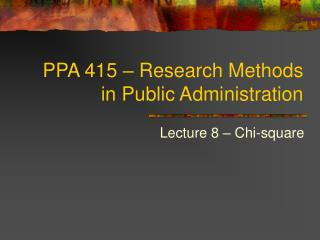# PPA 415 – Research Methods in Public Administration - PowerPoint PPT PresentationDownload PresentationPPA 415 – Research Methods in Public Administration

PPA 415 – Research Methods in Public AdministrationDownload Presentation## PPA 415 – Research Methods in Public Administration

- - - - - - - - - - - - - - - - - - - - - - - - - - - E N D - - - - - - - - - - - - - - - - - - - - - - - - - - -
##### Presentation Transcript

1. PPA 415 – Research Methods in Public Administration Lecture 8 – Chi-square

2. Introduction • The chi-square (2) has probably been the most frequently used test of hypothesis in the social sciences, largely because its assumptions are the easiest to satisfy. • It assumes only that the sample is randomly selected and the variable is measured at the nominal level. • No assumptions about shape of distribution or the sampling distribution.

3. Bivariate Tables • Bivariate tables are used to find if there is a significant relationship between the independent and dependent variable. • Rows (dependent variables), columns (independent variables), and cells (independent by dependent variable).

4. Bivariate Tables

5. The Logic of Chi-Square • In two-sample tests, independence means that the two samples are randomly selected. • In chi-square, independence means that the classification of a case on one variable has no influence on how cases are classified on the other variable.

6. The Logic of Chi-Square

7. The Computation of Chi-Square • Chi-square is calculated by comparing the observed frequency (fo) each cell to the expected frequency (fe)if there were no relationship. • The larger the difference between observed and expected frequency, the larger is chi-square.

8. The Computation of Chi-Square

9. The Computation of Chi-Square • Example:

10. Five-Step Hypothesis Test • Step 1. Making assumptions. • Independent random samples. • Nominal level of measurement. • Step 2. Stating the null hypothesis. • H0: The two variables are independent. • H1: The two variables are dependent (related).

11. Five-Step Hypothesis Test • Step 3. Selecting the sampling distribution and establishing the critical region. • Sampling distribution = 2 distribution. • Alpha=0.05. • Df=(r-1)(c-1)=(3-1)(2-1)=2(1)=2. • 2(critical)=Appendix C, p. 466=5.991.

12. Five-Step Hypothesis Test • Step 4. • 2(obtained)=5.87 (from slide 9). • Step 5. Making a decision. • 2(obtained) < 2(critical), therefore do not reject the null hypothesis that there is no relationship between presidential administration and disaster recommendations.

13. Example 2

14. Example 2 • Step 1. Making assumptions. • Independent random samples. • Nominal level of measurement. • Step 2. Stating the null hypothesis. • H0: The two variables are independent. • H1: The two variables are dependent (related).

15. Example 2 • Step 3. Selecting the sampling distribution and establishing the critical region. • Sampling distribution = 2 distribution. • Alpha=0.05. • Df=(r-1)(c-1)=(2-1)(3-1)=1(2)=2. • 2(critical)=Appendix C, p. 466=5.991.

16. Example 2 • Step 4.

17. Example 2 • Step 5. Making a decision. • 2(obtained) >2(critical), therefore reject the null hypothesis that there is no relationship between ethnicity and whether or not Birmingham residents have experienced discrimination. Minorities are significantly more likely to report that they have experienced discrimination than whites.

18. Limitations of Chi-Square • The chi-square becomes difficult to interpret if the table is larger than 4 x 4. • When sample size is small, expected frequencies can be less than five per cell. If the table is 2 x 2, use the following correction.

19. Limitations of Chi-Square • If table is more than 2 x 2, there is no correction for small sample size. • Chi-square is also sensitive to large sample sizes. For example, doubling the sample size, doubles chi-square. For large samples, very small (and meaningless) differences can be significant. You should also calculate a measure of association. See the next lecture.